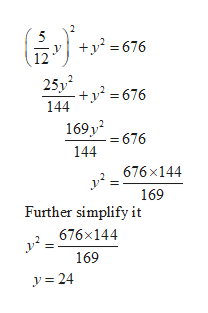# The ratio of two numbers is 5 to 12 and the sum of their squares is 676. Find the numbers.

Question
30 views

The ratio of two numbers is 5 to 12 and the sum of their squares is 676. Find the numbers.

check_circle

Step 1

Let us consider the numbers are x and y.

Since given the ratio of two numbers are 5 to 12, which is given below.

Step 2

And the sum of square is 676, which is given below

Step 3

In order to find the value of x and y...help_outlineImage Transcriptionclose+y? = 676 25y +y = 676 144 169y = 676 144 676x144 y?: 169 Further simplify it 676x144 169 y = 24 fullscreen

### Want to see the full answer?

See Solution

#### Want to see this answer and more?

Solutions are written by subject experts who are available 24/7. Questions are typically answered within 1 hour.*

See Solution
*Response times may vary by subject and question.
Tagged in

### Algebra